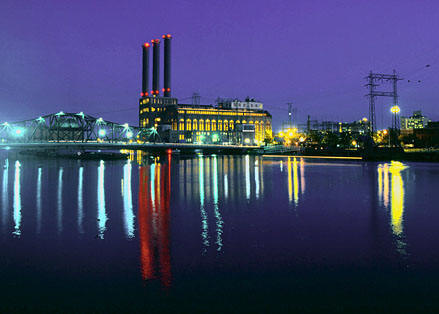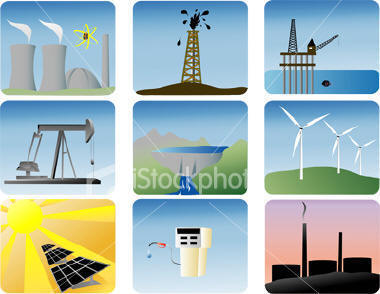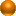Energy & Power plants engineering
Renewable energies: Solar Energy, Geothermal Energy, Wind Energy, Non-Renewable Energies: Fossil Fuels, Nuclear Energy, Biomass EnergyTypes of Energies

Renewable energy

Non-renewable energyEnter your search terms Submit search formEnergy:
Energy is the ability to do work. When we move something by pushing or pulling, we are doing work.
Energy is measured in joules (J). The work done, or energy used, during a certain time is called power.
Power is measured in watts (W). A watt is the use of one joule of energy for one second.
A watt is a small amount of energy so we measure power in units of 1000 watts for one hour - kilowatts for one hour.
Energy cannot be made or destroyed, but it can change from one form to another.
Energy takes various forms, such as; potential energy, (stored energy), kinetic energy, (movement energy), thermal, chemical, magnetic, electrical. etcSources of Energy:
Energy resources can be described as renewable and non-renewable.
Renewable energy sources are those which are continually being replaced such as energy from the sun (solar) and wind.
If an energy resource is being used faster than it can be replaced (for example, coal takes millions of years to form) then it will eventually run out.
This is called a non-renewable energy source.

Renewable energies: Solar Energy, Geothermal Energy, Wind Energy, Non-Renewable Energies: Fossil Fuels, Nuclear Energy, Biomass Energy